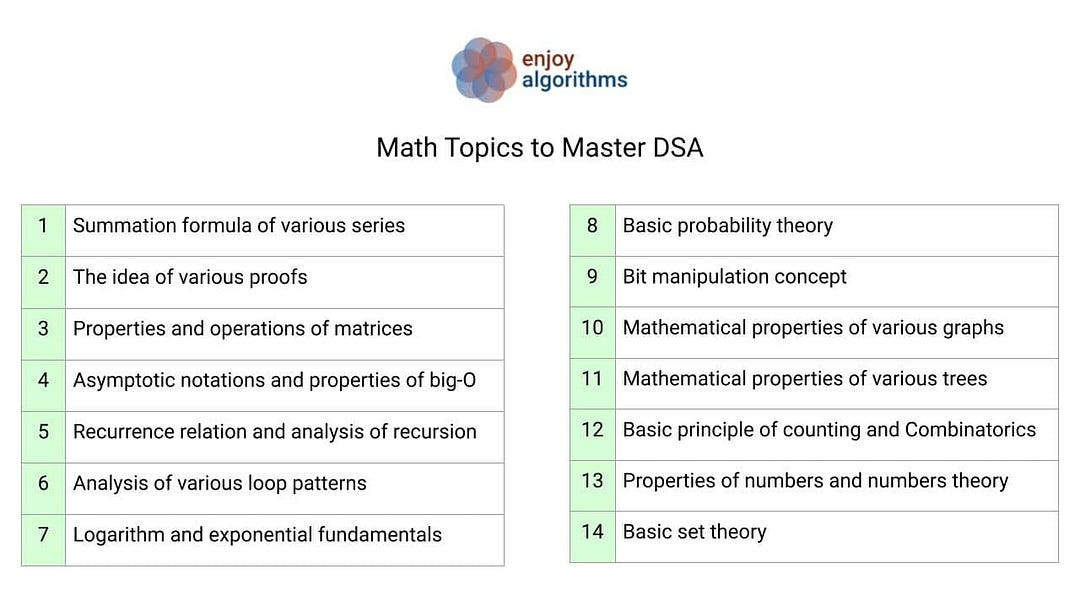# Important Math Topics for Data Structures and Algorithms

Just like programming, math is one of the core parts of learning data structures and algorithms. We mainly use math to analyse efficiency of various algorithms. But sometimes, the problem itself contains mathematical property or requires some mathematical insight to find a solution.

So learning math is crucial for mastering algorithms and data structures. The critical question is: What type of math or mathematical thinking is essential for DSA? Here is a comprehensive list of topics:Summation formula and properties of various series: Arithmetic series, Geometric series, Infinite geometric series, Harmonic series, Sum of squares and cubes, Telescopic series, Finding nth terms of different series, etc.

The idea of various proofs: Proof by contradiction, Proof by mathematical induction, Proof by logical deduction, Proof by contraposition, etc.

Properties and operations of matrices: Matrices addition, Matrices subtraction, Matrices multiplication, Transpose, Inverse, Types of matrices, Determinant of a matrix, Solving systems of linear equations using matrices, etc.

Analysis of recursion: Recurrence relation of various recursive functions, Substitution method, Recursion tree method, Master theorem

Analysis of various loop patterns: Single loop patterns, Two nested loop patterns, Three nested loop patterns, etc.

Combinatorics: Basic principle of counting, Permutations of sets, Combinations of sets, Permutations of multisets, Combinations of multisets, Decision problem, Exhaustive search problem, Optimization problem, The rule of product, The rule of sum, Permutations with repetition, Combinations with repetition, Pascal Triangle, Principle of inclusion-exclusion, Piegon hole principle, Catalan number, etc.

Properties of numbers and numbers theory: Various representations of numbers (Binary, decimal, hexadecimal, etc.), properties of natural and prime numbers, LCM, GCD, Square root, Factorial, Prime factorization, Primality test, Rule of divisibility, Properties of Fibonacci numbers, Modular arithmetic, Chinese remainder theorem, Fermat's theorem, etc.

Basic probability theory: Random Variables, Bayes’ Rule, Analysis of randomized algorithms, Conditional probability, Independence, Conditional independence, etc.

Bit manipulation, Operators and their various use cases: Bitwise AND, Bitwise OR, Bitwise NOT, Bitwise XOR, Left shift, Right shift, Bit Masking, etc.

Mathematical properties of various graph types: Directed graph, undirected graph, sparse graph, dense graph, bipartite graph, Directed acyclic graph, cycle, Connectivity, degree, path, subpath, Connected components, Properties of BFS and DFS, Graph colouring, etc.

Mathematical properties of various trees: Full binary tree, Complete binary tree, Perfect binary tree, Height balanced tree, BST property, Heap property, Segment tree, Trie, Decision tree, Fenwick tree, etc.

Other important concepts: Asymptotic notations, properties of big-O, Logarithm and exponential fundamentals, Basic set theory, etc.

We are planning to write a separate blog on each topic later. In the meantime, we are looking forward to your suggestions and critical feedback. Enjoy mathematics!

Share on social media:

© 2020 Code Algorithms Pvt. Ltd.